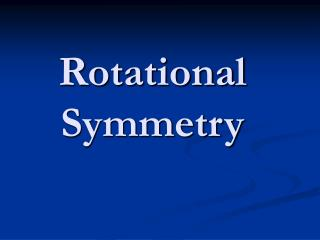DownloadDownload PresentationRotational Symmetry

# Rotational Symmetry

Download Presentation## Rotational Symmetry

- - - - - - - - - - - - - - - - - - - - - - - - - - - E N D - - - - - - - - - - - - - - - - - - - - - - - - - - -
##### Presentation Transcript

1. Rotational Symmetry

2. Rotational Symmetry • If, when you rotate a shape, it looks exactly the same as it did in its original position, then we say that the shape has got rotational symmetry. • This square has got rotational symmetry. • It looks exactly the same four times in a full turn. • It has got rotational symmetry order 4.

3. Rotational Symmetry • Does this rectangle have rotational symmetry? No, it doesn’t fit No, it doesn’t fit Yes, it fits! Yes, it fits! Click to rotate the rectangle. • It looks exactly the same twice in a full turn. • It has got rotational symmetry order 2.

4. Rotational Symmetry in the Alphabet Which letters have got rotational symmetry? Click to see the answers. A B C D E F G H I J Order 2 K L Order 2 M N Order 2 O P Q Order 2 R S T U V W X Y Z Order 2 Order 2 Order 2

5. Rotational Symmetry all around us Which road signs have got rotational symmetry? Click to see the answers.

6. Rotational Symmetry all around us Click to see the road signs with rotational symmetry. Order 3 Order 2 Order 2

7. Rotational Symmetry all around us Click to see more road signs with rotational symmetry. Order 4 Order 3 Order 2

8. Rotational Symmetry all around us Click to see more road signs with rotational symmetry. Order 3 Order 2

9. Rotational Symmetry with Pentominoes • If you don’t know what a pentomino is, then click here. • If you have done the pentominoes activity before, then you will know what a pentomino is and how many different pentominoes there are. • Here they are ...

10. Rotational Symmetry with Pentominoes Which Pentominoes have got rotational symmetry? Sort them according to their rotational symmetry.

11. Rotational Symmetry with Pentominoes Rotational Symmetry Order 2 Rotational Symmetry Order 4 Click to start sorting

12. Rotational Symmetry with 7-Pin Polygons • If you don’t know what a 7-pin polygon is, then click here. • If you have done the 7-pin polygons activity before, then you will know what a 7-pin polygon is and how many different ones there are. • Here they are ...

13. Rotational Symmetry with 7-Pin Polygons Which 7-Pin Polygons have got rotational symmetry? Sort them according to their rotational symmetry.

14. Rotational Symmetry with 7-Pin Polygons Rotational Symmetry Order 2 Rotational Symmetry Order 3 Rotational Symmetry Order 6 Click to start sorting

15. Pentominoes A pentomino is made out of five squares that are connected along at least one edge. Here is an example of a pentomino. These are NOT pentominoes. How many different pentominoes can you find? Click here to return to line symmetry.

16. 7-Pin Polygons Here is an arrangement of 7 pins. A 7-pin polygon is a closed shape with straight sides with corners on the pins. Here are some examples of 7-pin polygons. How many different 7-pin polygons can you find? Click here to return to line symmetry.

17. END OF PRESENTATION